# OpenGL Sphere

This page describes how to generate various spherical geometries using C++ and how to draw them with OpenGL.

### Sphere

The definition of sphere is a 3D closed surface where every point on the sphere is same distance (radius) from a given point. The equation of a sphere at the origin is.

Since we cannot draw all the points on a sphere, we only sample a limited amount of points by dividing the sphere by sectors (longitude) and stacks (latitude). Then connect these sampled points together to form surfaces of the sphere.Sectors and stacks of a sphereA point on a sphere using sector and stack angles

An arbitrary point (x, y, z) on a sphere can be computed by parametric equations with the corresponding sector angle θ and stack angle ϕ.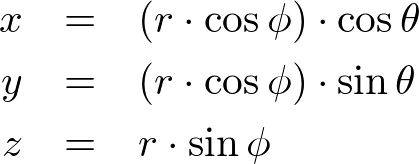The range of sector angles is from 0 to 360 degrees, and the stack angles are from 90 (top) to -90 degrees (bottom). The sector and stack angle for each step can be calculated by the following;The following C++ code generates all vertices of the sphere for the given radius, sectors and stacks. It also creates other vertex attributes; surface normals and texture coordinates. For more details, please refer to buildVerticesSmooth() or buildVerticesFlat() functions in Sphere.cpp class.

``````
// clear memory of prev arrays
std::vector<float>().swap(vertices);
std::vector<float>().swap(normals);
std::vector<float>().swap(texCoords);

float x, y, z, xy;                              // vertex position
float nx, ny, nz, lengthInv = 1.0f / radius;    // vertex normal
float s, t;                                     // vertex texCoord

float sectorStep = 2 * PI / sectorCount;
float stackStep = PI / stackCount;
float sectorAngle, stackAngle;

for(int i = 0; i <= stackCount; ++i)
{
stackAngle = PI / 2 - i * stackStep;        // starting from pi/2 to -pi/2
xy = radius * cosf(stackAngle);             // r * cos(u)
z = radius * sinf(stackAngle);              // r * sin(u)

// add (sectorCount+1) vertices per stack
// the first and last vertices have same position and normal, but different tex coords
for(int j = 0; j <= sectorCount; ++j)
{
sectorAngle = j * sectorStep;           // starting from 0 to 2pi

// vertex position (x, y, z)
x = xy * cosf(sectorAngle);             // r * cos(u) * cos(v)
y = xy * sinf(sectorAngle);             // r * cos(u) * sin(v)
vertices.push_back(x);
vertices.push_back(y);
vertices.push_back(z);

// normalized vertex normal (nx, ny, nz)
nx = x * lengthInv;
ny = y * lengthInv;
nz = z * lengthInv;
normals.push_back(nx);
normals.push_back(ny);
normals.push_back(nz);

// vertex tex coord (s, t) range between [0, 1]
s = (float)j / sectorCount;
t = (float)i / stackCount;
texCoords.push_back(s);
texCoords.push_back(t);
}
}

``````vertex indices to draw triangles of a sphere

In order to draw the surface of a sphere in OpenGL, you must triangulate adjacent vertices to form polygons. It is possible to use a single triangle strip to render the whole sphere. However, if the shared vertices have different normals or texture coordinates, then a single triangle strip cannot be used.

Each sector in a stack requires 2 triangles. If the first vertex index in the current stack is k1 and the next stack is k2, then the counterclockwise orders of vertex indices of 2 triangles are;
k1 ⟶ k2 ⟶ k1+1
k1+1 ⟶ k2 ⟶ k2+1

But, the top and bottom stacks require only one triangle per sector. The code snippet to generate all triangles of a sphere may look like;

``````
// generate CCW index list of sphere triangles
// k1--k1+1
// |  / |
// | /  |
// k2--k2+1
std::vector<int> indices;
std::vector<int> lineIndices;
int k1, k2;
for(int i = 0; i < stackCount; ++i)
{
k1 = i * (sectorCount + 1);     // beginning of current stack
k2 = k1 + sectorCount + 1;      // beginning of next stack

for(int j = 0; j < sectorCount; ++j, ++k1, ++k2)
{
// 2 triangles per sector excluding first and last stacks
// k1 => k2 => k1+1
if(i != 0)
{
indices.push_back(k1);
indices.push_back(k2);
indices.push_back(k1 + 1);
}

// k1+1 => k2 => k2+1
if(i != (stackCount-1))
{
indices.push_back(k1 + 1);
indices.push_back(k2);
indices.push_back(k2 + 1);
}

// store indices for lines
// vertical lines for all stacks, k1 => k2
lineIndices.push_back(k1);
lineIndices.push_back(k2);
if(i != 0)  // horizontal lines except 1st stack, k1 => k+1
{
lineIndices.push_back(k1);
lineIndices.push_back(k1 + 1);
}
}
}

``````

### Example: Sphere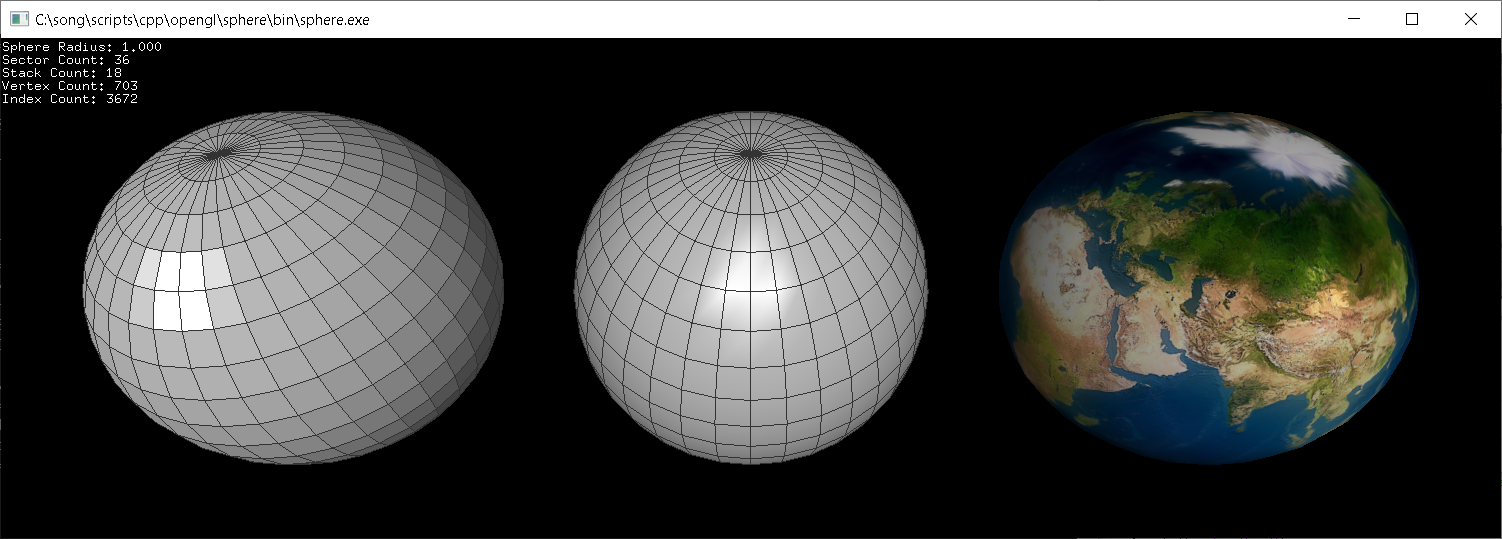Download: sphere.zip, sphereShader.zip (Updated: 2020-05-20)

This example constructs spheres with 36 sectors and 18 stacks, but with different shadings; flat, smooth or textured. Sphere.cpp class provides pre-defined functions; draw(), drawWithLines() and drawLines(), to draw a sphere using OpenGL VertexArray.

``````
// create a sphere with radius=1, sectors=36, stacks=18, smooth=true (default)
Sphere sphere(1.0f, 36, 18);

// can change parameters later
sphere.setRadius(2.0f);
sphere.setSectorCount(72);
sphere.setStackCount(24);
sphere.setSmooth(false);
...

// draw sphere using vertexarray
sphere.draw();

``````

This C++ class also provides getVertices(), getIndices(), getInterleavedVertices(), etc. in order to access the vertex data in GLSL. The following code draws a sphere with interleaved vertex data using VBO and GLSL. Or, download sphereShader.zip for more details.

``````
// create a sphere with default params; radius=1, sectors=36, stacks=18, smooth=true
Sphere sphere;

// copy interleaved vertex data (V/N/T) to VBO
GLuint vboId;
glGenBuffers(1, &vboId);
glBindBuffer(GL_ARRAY_BUFFER, vboId);           // for vertex data
glBufferData(GL_ARRAY_BUFFER,                   // target
sphere.getInterleavedVertexSize(), // data size, # of bytes
sphere.getInterleavedVertices(),   // ptr to vertex data
GL_STATIC_DRAW);                   // usage

// copy index data to VBO
GLuint iboId;
glGenBuffers(1, &iboId);
glBindBuffer(GL_ELEMENT_ARRAY_BUFFER, iboId);   // for index data
glBufferData(GL_ELEMENT_ARRAY_BUFFER,           // target
sphere.getIndexSize(),             // data size, # of bytes
sphere.getIndices(),               // ptr to index data
GL_STATIC_DRAW);                   // usage
...

// bind VBOs
glBindBuffer(GL_ARRAY_BUFFER, vboId);
glBindBuffer(GL_ELEMENT_ARRAY_BUFFER, iboId);

// activate attrib arrays
glEnableVertexAttribArray(attribVertex);
glEnableVertexAttribArray(attribNormal);
glEnableVertexAttribArray(attribTexCoord);

// set attrib arrays with stride and offset
int stride = sphere.getInterleavedStride();     // should be 32 bytes
glVertexAttribPointer(attribVertex,   3, GL_FLOAT, false, stride, (void*)0);
glVertexAttribPointer(attribNormal,   3, GL_FLOAT, false, stride, (void*)(sizeof(float)*3));
glVertexAttribPointer(attribTexCoord, 2, GL_FLOAT, false, stride, (void*)(sizeof(float)*6));

// draw a sphere with VBO
glDrawElements(GL_TRIANGLES,                    // primitive type
sphere.getIndexCount(),          // # of indices
GL_UNSIGNED_INT,                 // data type
(void*)0);                       // offset to indices

// deactivate attrib arrays
glDisableVertexAttribArray(attribVertex);
glDisableVertexAttribArray(attribNormal);
glDisableVertexAttribArray(attribTexCoord);

// unbind VBOs
glBindBuffer(GL_ARRAY_BUFFER, 0);
glBindBuffer(GL_ELEMENT_ARRAY_BUFFER, 0);

``````

Since this C++ class uses cylindrical texture mapping, there is a squeeze/distortion at the north and south pole area. This problem can be solved using Icosphere or Cubesphere.

### Icosphere / Geosphere

Another way to create a spherical geometry is subdividing an icosahedron multiple times. Icosahedron is a regular polyhedron with 12 vertices and 20 equilateral triangles (the first left image below). Each triangle of an icosahedron is divided into 4 equal sub-triangles per subdivision.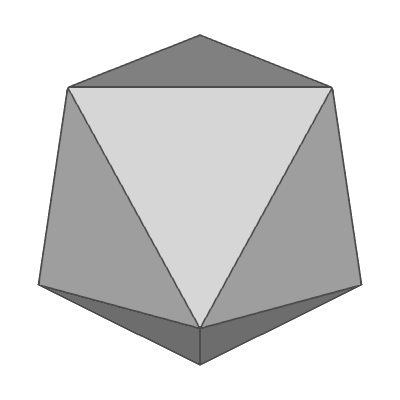Icosphere at subdivision 0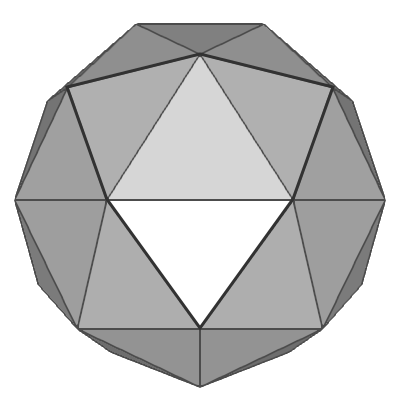Icosphere at subdivision 1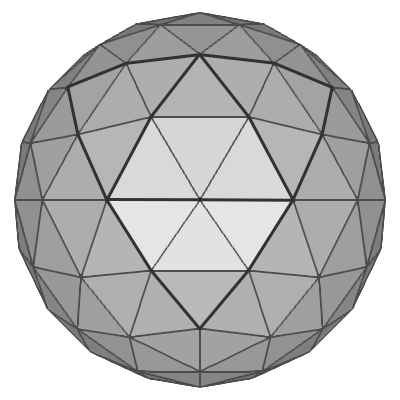Icosphere at subdivision 2Icosphere at subdivision 3

One way to construct 12 vertices of an icosahedron is using spherical coordinates; aligning 2 vertices at the north and south poles, and the other 10 vertices are placed at latitude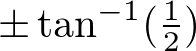degrees and 72° aside on the same latitude. Please see the following orthogonal projection images of icosahedron.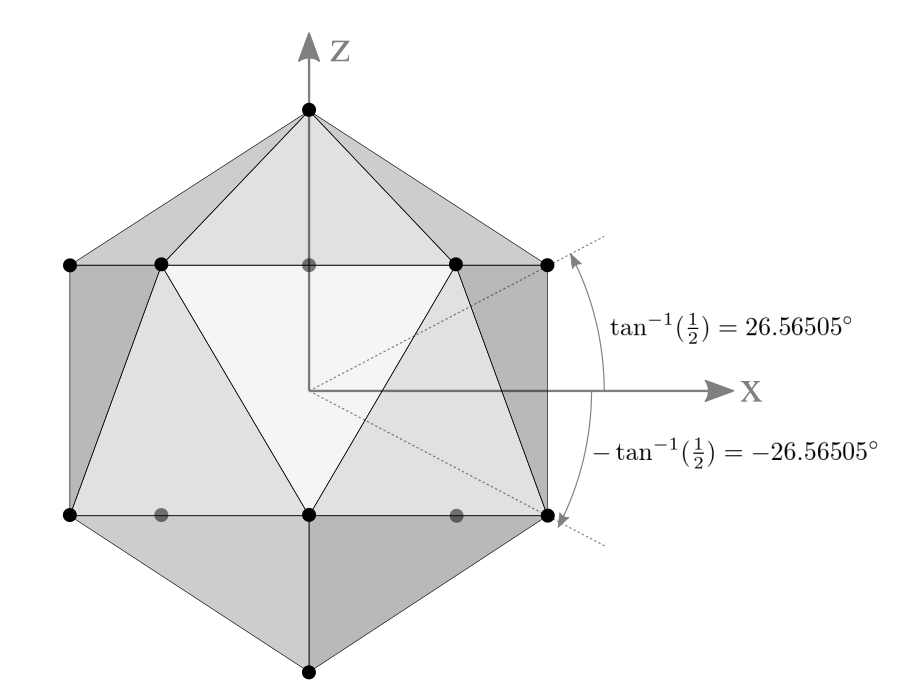Side View of Icosahedron
2 vertices are at north/south pole and 10 vertices are at elevation ±26.565°Top View of Icosahedron
5 vertices are 72° apart at the same elevation

A typical point at latitude 26.565° and radius r can be computed by;Note thatis the elevation (height) of the point andis the length of the projected line segment on XY plane.
(Reference: Spherical Coordinates of Regular Icosahedron from Wikipedia)

The following C++ code is to generate 12 vertices of an icosahedron for a given radius, or you can find the complete implementation of Icosahedron.cpp or Icosphere.cpp class.

``````
// constants
const float PI = 3.1415926f;
const float H_ANGLE = PI / 180 * 72;    // 72 degree = 360 / 5
const float V_ANGLE = atanf(1.0f / 2);  // elevation = 26.565 degree

std::vector<float> vertices(12 * 3);    // array of 12 vertices (x,y,z)
int i1, i2;                             // indices
float z, xy;                            // coords
float hAngle1 = -PI / 2 - H_ANGLE / 2;  // start from -126 deg at 1st row
float hAngle2 = -PI / 2;                // start from -90 deg at 2nd row

// the first top vertex at (0, 0, r)
vertices = 0;
vertices = 0;
vertices = radius;

// compute 10 vertices at 1st and 2nd rows
for(int i = 1; i <= 5; ++i)
{
i1 = i * 3;         // index for 1st row
i2 = (i + 5) * 3;   // index for 2nd row

z  = radius * sinf(V_ANGLE);            // elevaton
xy = radius * cosf(V_ANGLE);            // length on XY plane

vertices[i1] = xy * cosf(hAngle1);      // x
vertices[i2] = xy * cosf(hAngle2);
vertices[i1 + 1] = xy * sinf(hAngle1);  // y
vertices[i2 + 1] = xy * sinf(hAngle2);
vertices[i1 + 2] = z;                   // z
vertices[i2 + 2] = -z;

// next horizontal angles
hAngle1 += H_ANGLE;
hAngle2 += H_ANGLE;
}

// the last bottom vertex at (0, 0, -r)
i1 = 11 * 3;
vertices[i1] = 0;
vertices[i1 + 1] = 0;
vertices[i1 + 2] = -radius;

``````

The subdivision algorithm is splitting the 3 edge lines of each triangle in half, then extruding the new middle point outward, so its length (the distance from the center) is the same as sphere's radius.

``````
std::vector<float> tmpVertices;
std::vector<float> tmpIndices;
const float *v1, *v2, *v3;          // ptr to original vertices of a triangle
float newV1, newV2, newV3; // new vertex positions
unsigned int index;

// iterate all subdivision levels
for(int i = 1; i <= subdivision; ++i)
{
// copy prev vertex/index arrays and clear
tmpVertices = vertices;
tmpIndices = indices;
vertices.clear();
indices.clear();
index = 0;

// perform subdivision for each triangle
for(int j = 0; j < tmpIndices.size(); j += 3)
{
// get 3 vertices of a triangle
v1 = &tmpVertices[tmpIndices[j] * 3];
v2 = &tmpVertices[tmpIndices[j + 1] * 3];
v3 = &tmpVertices[tmpIndices[j + 2] * 3];

// compute 3 new vertices by spliting half on each edge
//         v1
//        / \
// newV1 *---* newV3
//      / \ / \
//    v2---*---v3
//       newV2
computeHalfVertex(v1, v2, newV1);
computeHalfVertex(v2, v3, newV2);
computeHalfVertex(v1, v3, newV3);

// add 4 new triangles to vertex array
addVertices(v1,    newV1, newV3);
addVertices(newV1, v2,    newV2);
addVertices(newV1, newV2, newV3);
addVertices(newV3, newV2, v3);

// add indices of 4 new triangles
addIndices(index,   index+1, index+2);
addIndices(index+3, index+4, index+5);
addIndices(index+6, index+7, index+8);
addIndices(index+9, index+10,index+11);
index += 12;    // next index
}
}

///////////////////////////////////////////////////////////////////////////////
// find middle point of 2 vertices
// NOTE: new vertex must be resized, so the length is equal to the radius
///////////////////////////////////////////////////////////////////////////////
void computeHalfVertex(const float v1, const float v2, float newV)
{
newV = v1 + v2;    // x
newV = v1 + v2;    // y
newV = v1 + v2;    // z
float scale = radius / sqrtf(newV*newV + newV*newV + newV*newV);
newV *= scale;
newV *= scale;
newV *= scale;
}

``````

In order to generate a texture map of an icosphere, you need to unwrap the 3D geometry on a plane (paper model). I use the following texture coordinates of vertices instead of normalized coordinates from 0 to 1, so the coordinate of each vertex can be snapped to an exact pixel on the image. For example, if a texture size is 2048x1024, then the horizontal step is 186 pixels and the vertical step is 322 pixels.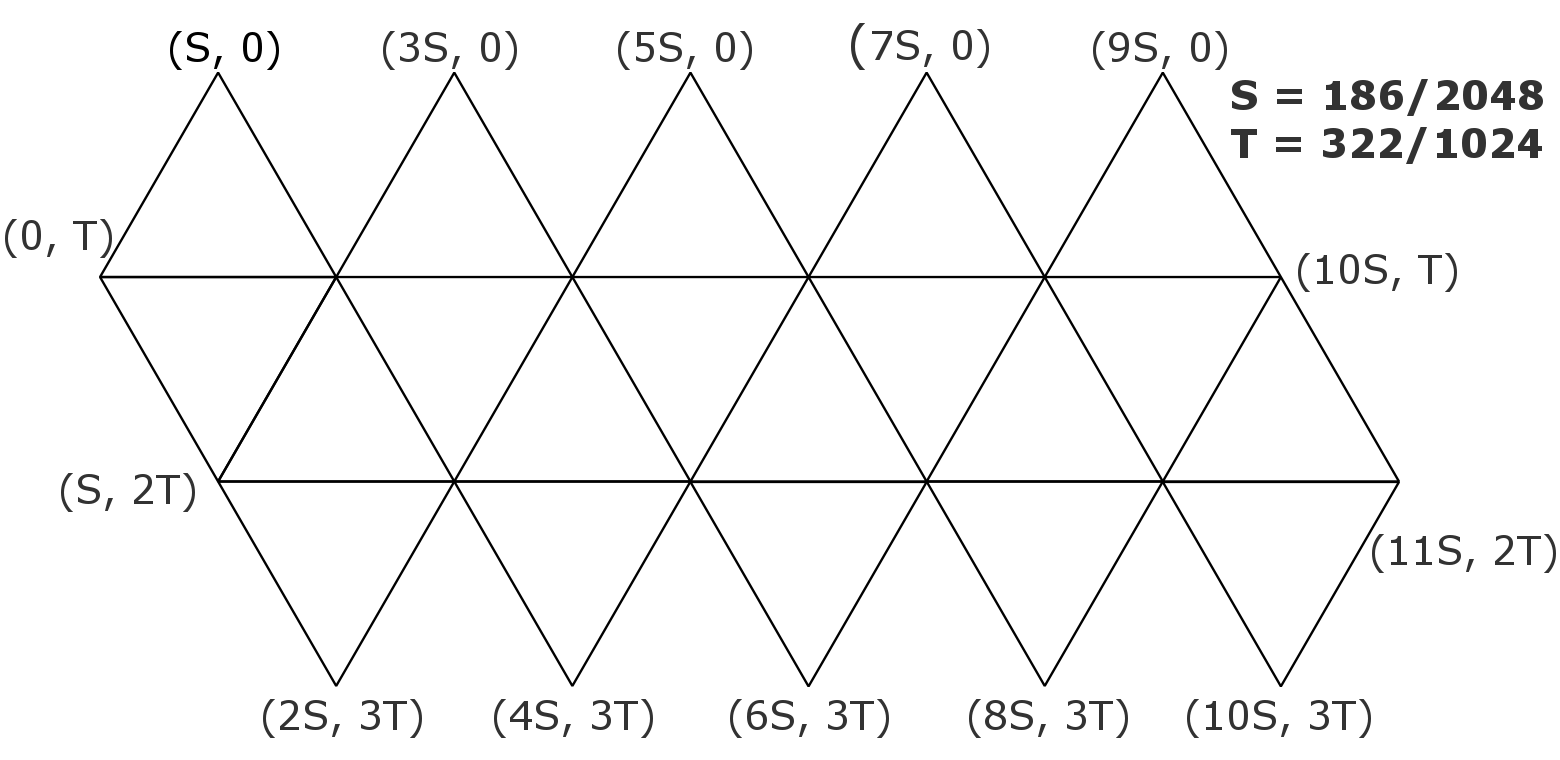Texture coordinates of Icosphere, where S=186/2048, T=322/1024

### Example: Icosphere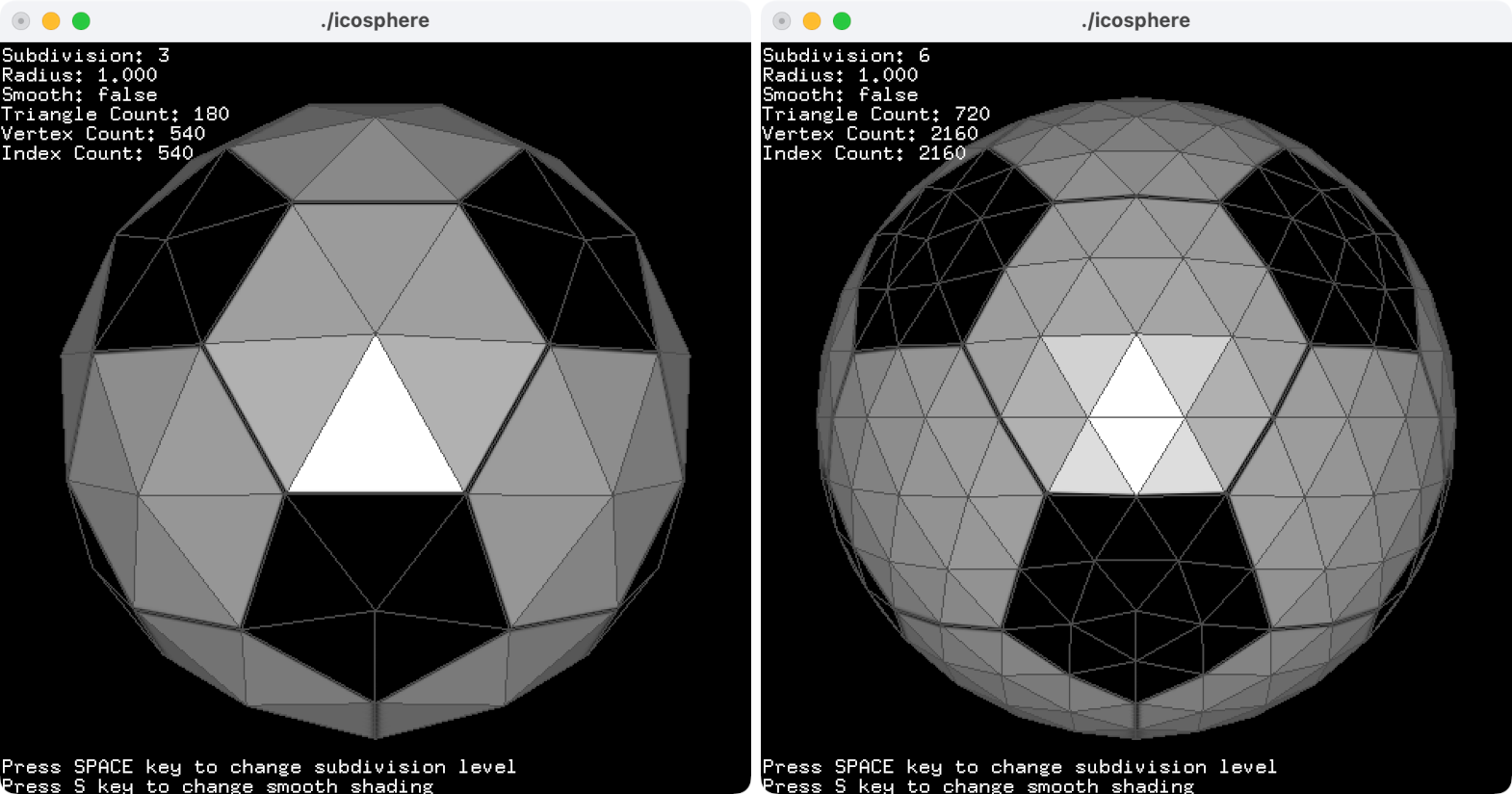This example is to draw an icosphere with a texture. Press the spacebar key to change the subdivision level. If the subdivision is 2, the icosphere consists of 320 triangles, and if the subdivision is 5, it has 20,480 triangles.
Download: icosphere.zip, icosphereShader.zip, icosahedron.zip (Updated: 2019-12-29)

Drawing an icosphere in OpenGL is identical to Sphere C++ class object. Please refer to Sphere example section above. To construct an icosphere object, it requires 3 parameters; radius, subdivision and surface smoothness. You can change the radius and subdivision level after it has been constructed. If the subdivision is 0, then it is the same as an icosahedron.

``````
// create icosphere with radius=1, subdivision=5 and smooth shading=true
Icosphere sphere(1.0f, 5, true);

// can change parameters later
sphere.setRadius(2.0f);
sphere.setSubdivision(6);
sphere.setSmooth(false);
...

// draw icosphere using vertexarray
sphere.draw();
...

// copy interleaved vertex data (V/N/T) to VBO
GLuint vboId;
glGenBuffers(1, &vboId);
glBindBuffer(GL_ARRAY_BUFFER, vboId);           // for vertex data
glBufferData(GL_ARRAY_BUFFER,                   // target
sphere.getInterleavedVertexSize(), // data size, # of bytes
sphere.getInterleavedVertices(),   // ptr to vertex data
GL_STATIC_DRAW);                   // usage

// copy index data to VBO
GLuint iboId;
glGenBuffers(1, &iboId);
glBindBuffer(GL_ELEMENT_ARRAY_BUFFER, iboId);   // for index data
glBufferData(GL_ELEMENT_ARRAY_BUFFER,           // target
sphere.getIndexSize(),             // data size, # of bytes
sphere.getIndices(),               // ptr to index data
GL_STATIC_DRAW);                   // usage
...

// draw icosphere using VBO and GLSL
int stride = sphere.getInterleavedStride();     // should be 32 bytes
glVertexAttribPointer(attribVertex,   3, GL_FLOAT, false, stride, (void*)0);
glVertexAttribPointer(attribNormal,   3, GL_FLOAT, false, stride, (void*)(sizeof(float)*3));
glVertexAttribPointer(attribTexCoord, 2, GL_FLOAT, false, stride, (void*)(sizeof(float)*6));
glDrawElements(GL_TRIANGLES,
sphere.getIndexCount(),
GL_UNSIGNED_INT,
(void*)0);
...

``````

### Cubesphere

Similar to subdividing an icosahedron, a cubesphere is subdividing a cube (a regular polyhedron with 6 faces) N times to construct a sphere geometry. The characteristic of a cubesphere is that the spherical surface of the sphere is decomposed into 6 equal-area regions (+X, -X, +Y, -Y, +Z and -Z faces). It is somewhat related to the cube map, which is a method of environment mapping in computer graphics.Cubesphere at subdivision 0Cubesphere at subdivision 1Cubesphere at subdivision 2Cubesphere at subdivision 3

The following images represent how to construct one of the 6 regions of a cubesphere by intersecting angularly equal-distant longitudinal and latitudinal lines from -45 degree to 45 degree. A vertex on a cubesphere can be computed by the intersection of 2 plane equations. If the normal vector of the latitudinal plane is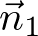and the normal of the longitudinal plane is, then the direction vector of the intersect line is. Finally, the vertex on the cubesphere is scaling the normalized direction vector by the radius of the sphere,.A face of cubesphere by intersecting longitudinal and latitudinal lines from -45 to +45 degree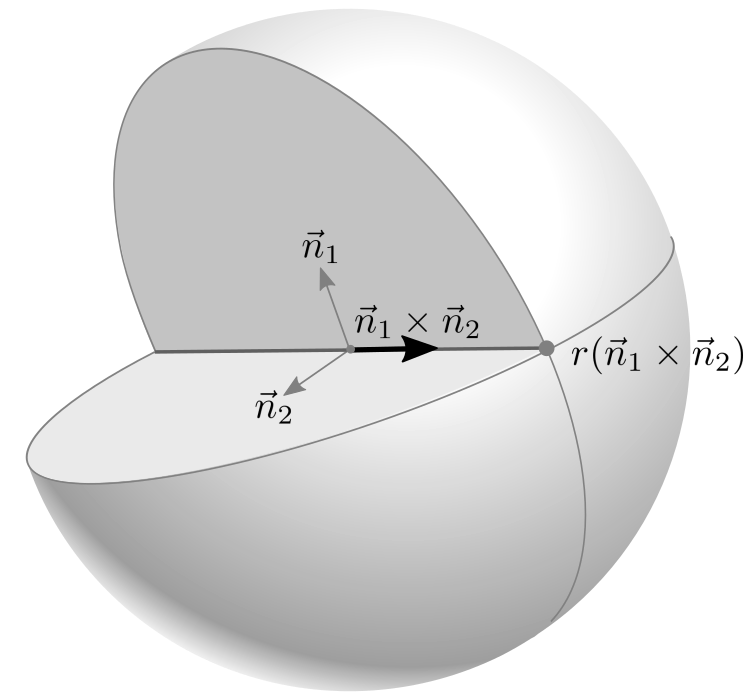An intersect point of latitudinal and longitudinal planes

The following C++ code is generating a face of a unit cubesphere, which is facing +X axis and the radius is 1.

``````
// generate vertices for +X face only by intersecting 2 circular planes
// (longitudinal and latitudinal) at the given longitude/latitude angles
std::vector<float> buildUnitPositiveX(int subdivision)
{
const float DEG2RAD = acos(-1) / 180.0f;

std::vector<float> vertices;
float n1;        // normal of longitudinal plane rotating along Y-axis
float n2;        // normal of latitudinal plane rotating along Z-axis
float v;         // direction vector intersecting 2 planes, n1 x n2
float a1;           // longitudinal angle along Y-axis
float a2;           // latitudinal angle along Z-axis

// compute the number of vertices per row, 2^n + 1
int pointsPerRow = (int)pow(2, subdivision) + 1;

// rotate latitudinal plane from 45 to -45 degrees along Z-axis (top-to-bottom)
for(unsigned int i = 0; i < pointsPerRow; ++i)
{
// normal for latitudinal plane
// if latitude angle is 0, then normal vector of latitude plane is n2=(0,1,0)
// therefore, it is rotating (0,1,0) vector by latitude angle a2
a2 = DEG2RAD * (45.0f - 90.0f * i / (pointsPerRow - 1));
n2 = -sin(a2);
n2 = cos(a2);
n2 = 0;

// rotate longitudinal plane from -45 to 45 along Y-axis (left-to-right)
for(unsigned int j = 0; j < pointsPerRow; ++j)
{
// normal for longitudinal plane
// if longitude angle is 0, then normal vector of longitude is n1=(0,0,-1)
// therefore, it is rotating (0,0,-1) vector by longitude angle a1
a1 = DEG2RAD * (-45.0f + 90.0f * j / (pointsPerRow - 1));
n1 = -sin(a1);
n1 = 0;
n1 = -cos(a1);

// find direction vector of intersected line, n1 x n2
v = n1 * n2 - n1 * n2;
v = n1 * n2 - n1 * n2;
v = n1 * n2 - n1 * n2;

// normalize direction vector
float scale = 1 / sqrt(v*v + v*v + v*v);
v *= scale;
v *= scale;
v *= scale;

// add a vertex into array
vertices.push_back(v);
vertices.push_back(v);
vertices.push_back(v);
}
}

return vertices;
}

``````

The other 5 faces can be generated by repeating the above procedure, or swapping and/or negating axis of the vertices of +X face to optimize redundant sine/cosine computations. For example, the vertices of -X face are only negating x and z coordinates of +X face, and +Y face requires swapping x → y, y → -z, and z → -x.

Please see the detail implementation in buildVerticesFlat() and buildVerticesSmooth() of C++ Cubesphere class to construct all 6 faces.

### Example: CubesphereDownload: cubesphere.zip, cubesphereShader.zip (Updated: 2020-01-08)

This example is to draw cubespheres with various shadings; the left sphere is without texture, the center sphere is applying a 2D texture to all the 6 faces, and the right sphere is with a cube map texture (GL_ARB_texture_cube_map extension required). Press the space key to change the subdivision levels.

To construct a cubesphere object, it requires 3 parameters; radius, subdivision and surface smoothness. You can change the radius and subdivision level after it has been constructed. If the subdivision is 0, then the shape is a cube.

``````
// create cubesphere with default constructor
// radius=1, subdivision=3 and smooth shading=true
Cubesphere sphere;
Cubesphere sphere(1, 3, true); // same as above

// can change parameters later
sphere.setRadius(2.0f);
sphere.setSubdivision(6);
sphere.setSmooth(false);
...

// draw cubesphere using vertexarray
sphere.draw();          // draw surface only
sphere.drawWithLines(); // draw surface and lines
sphere.drawFace(0);     // draw only +X face, face index:0~5
...

``````

This C++ class provides an additional function, drawFace() to draw only the selected face for the given face index. The valid face index is 0 to 5; +X, -X, +Y, -Y, +Z, -Z face respectively.

### Example: WebGL Sphere (Interactive Demo)

Radius
Sector Count
Stack Count

It is a JavaScript version of Sphere class, Sphere.js, and rendering it with WebGL. Drag the sliders to change the parameters of the sphere. The fullscreen version is available here.

The following JavaScript code is to create and to render a sphere object.

``````
// create a sphere with 4 params: radius, sectors, stacks, smooth
let sphere = new Sphere(1, 36, 18, false);
...

// change params of sphere later
sphere.setRadius(2);
sphere.setSectorCount(8);
sphere.setStackCount(4);
sphere.setSmooth(true);
...

// draw a sphere with interleaved mode
gl.bindBuffer(gl.ARRAY_BUFFER, sphere.vboVertex);
gl.vertexAttribPointer(gl.program.attribPosition, 3, gl.FLOAT, false, sphere.stride, 0);
gl.vertexAttribPointer(gl.program.attribNormal, 3, gl.FLOAT, false, sphere.stride, 12);
gl.vertexAttribPointer(gl.program.attribTexCoord0, 2, gl.FLOAT, false, sphere.stride, 24);

gl.bindBuffer(gl.ELEMENT_ARRAY_BUFFER, sphere.vboIndex);
gl.drawElements(gl.TRIANGLES, sphere.getIndexCount(), gl.UNSIGNED_SHORT, 0);
...

``````
comments powered by Disqus